Courses

# Test: Functions- 1

## 15 Questions MCQ Test Quantitative Aptitude for SSC CGL | Test: Functions- 1

Description
This mock test of Test: Functions- 1 for GMAT helps you for every GMAT entrance exam. This contains 15 Multiple Choice Questions for GMAT Test: Functions- 1 (mcq) to study with solutions a complete question bank. The solved questions answers in this Test: Functions- 1 quiz give you a good mix of easy questions and tough questions. GMAT students definitely take this Test: Functions- 1 exercise for a better result in the exam. You can find other Test: Functions- 1 extra questions, long questions & short questions for GMAT on EduRev as well by searching above.
QUESTION: 1

Solution:
QUESTION: 2

Solution:
QUESTION: 3

### \$x = 6x +4 and £x = 8x – 2 Find the value of x for which \$x = £x?

Solution:
QUESTION: 4

If f(x) = 3x + 6, then what is the value of f (2) + f(7)?

Solution:
QUESTION: 5

A polynomial function P(x) is defined as,

P(x) = 4x3 – 2x2

If P (z -2) =0 & z ≠ 2, find the value of z?

Solution:
QUESTION: 6

For which of the following functions is f(ab) = f(a) * f(b)?

1. f(x) = x2
2. f (x) = √x
3. f (x) = 2x
Solution:
QUESTION: 7

If f(x) = 3x2 – 5x + 9 and g(x) = 4x – 5, then find the value of g( f(x)) at x = 3.

Solution:

The information provided in the question is:

f(x) = 3x2 – 5x + 9

g(x) = 4x – 5

We have to find out the value of g( f(x)) at x = 3.

f(x) = 3x2 – 5x + 9

f(3) = 3*(3)2 – 5*3 + 9

= 27 – 15 + 9

= 21

g(x) = 4x – 5

g(f(x)) = 4f(x) -5

g( f(3)) = 4f(3) – 5

= 4*21 – 5

= 84 – 5

= 79

QUESTION: 8

If v* = (1/v), find the value of

v* + (v*)* + ((v*)*)* if v = 1/2.

Solution:

Step 1: Question statement and Inferences

A function has been defined called v* such that v* = 1v

Step 2 & 3: Finding required values and calculating the final answer

v = 1/2

=> v*=1v=2

=> (v*)* = (2)* = 1/2

=> ((v*)*)* = (1/2)* = 2

=> v* + (v*)* + ((v*)*)* = 2 + 1/2 + 2 = 9/2.

QUESTION: 9

If # and \$ are mathematical operations such that p # q = (p+ q)2 and p \$ q = (p – q)2, what is the value of  (2 # 2) + (3 \$ 3) – (5 # 5) + (6 \$ 6)?

Solution:

We are given that

p # q = (p+ q)2 and

p \$ q = (p – q)2

To calculate the value of the whole expression, we need to calculate the values of the individual expressions:

(2 # 2) = (2 + 2)2 = 42 = 16

(3 \$ 3)  = (3 – 3)2 = 0

(5 # 5) = (5 + 5)2 = 100

(6 \$ 6) = (6 – 6)2 = 0

So,

(2 # 2) + (3 \$ 3) – (5 # 5) + (6 \$ 6) = 16 – 0 – 100 – 0 = -84

QUESTION: 10

A function is defined as f (n) = the number of factors of n. If f (p*q*r) = 8, where p, q and r are prime numbers, what is the value of p?

(1) p + q + r is an even number

(2) q < p < r

Solution:

Steps 1 & 2: Understand Question and Draw Inferences

We are given that:

f(n) = the number of factors of n and

f(p*q*r) = 8

This means,

Number of factors of pqr = 8

We have learnt that in order to find the total number of factors of any number X, we should write it in terms of its prime factors:

X = P1m * P2n * P3r . . . where P1, P2, P3 . . .  are the prime factors of X and m, n, r . . . are non-negative integers

The total number of prime factors of X = (m+1)(n+1)(r+1) . . .

Here, it is given that:

Total number of factors of pqr = 8

Now, 8 = 23 = (1+1)(1+1)(1+1)

Thus, total number of factors of pqr = (1+1)(1+1)(1+1)

Comparing this with the above formula, we can deduce that p, q and r are distinct prime numbers.

[Note: We can deduce that p, q and r are distinct prime numbers because if say, p was equal to r, in that case, we could write:

pqr = p2q

The total number of factors in this case would be = (2+1)(1+1) = 3*2 = 6.

Since we are given the total number of factors is not 6, we can say confidently that no two prime numbers are equal to one another]

We know that except 2, all the prime numbers are odd. 2 is the only even prime number.

Step 3: Analyze Statement 1

Statement (1) says:

p + q + r = even number.

Now, the sum of three integers can be an even number only if:

1. Either all the integers are even numbers.                     (Think 2 + 4 + 6 = 12)
2. Or one of them is even and two are odd numbers.     (Think 2 + 3 + 5 = 10)

Since p, q, and r are distinct prime numbers, all three of them can’t be even because there is only one even prime number.

So, the only possible condition is that one of p, q, and r is even number and the other two are odd numbers.

So, this statement tells us that the value of one of the numbers p, q, and r is 2. However, it does not tell us what the value of p is.

To conclude

One of p, q, or r is equal to 2.

So, statement (1) alone is not sufficient to answer the question “what is the value of p”.

Step 4: Analyze Statement 2

Statement (2) tells us that q < p < r. So, we know that the prime number p is greater than q and smaller than r.

However, since there are infinite number of prime numbers possible, we still cannot determine the value of p.

To conclude

q < p < r

Thus, statement (2) alone is not sufficient to answer the question “what is the value of p”.

Step 5: Analyze Both Statements Together (if needed)

Since the individual analysis of the choices does not tell us the value of p, let’s analyse both the statements together.

From statement (1): One of p, q, or r is equal to 2.

From statement (2): q < p < r

Now, since 2 is the smallest prime number, the value of q is 2. So, p is a prime number greater than 2, but we still don’t know the value of p.

So, analyzing both the statements together is not enough to determine the value of p.

QUESTION: 11

If x ≠ 0, for which of following functions is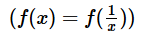, for all values of x?

Solution:

Step 1: Question statement and Inferences

We have to pick the only option for which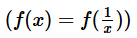, Since the option will have to be true for all the values of x, substituting numbers is not the best approach. We shall try to replace x with 1/x in each option.

Step 2 & 3: Finding required values and finding the final answer

Let’s check each option one by one.

A.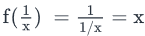.

Not equal to f(x)

B.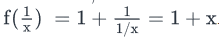Not equal to f(x)

C.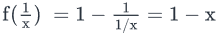.  Not equal to f(x)

D.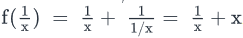Not equal to f(x)

In the exam, you need not evaluate option (E) – since we already know that Option D is the answer- but here for practice, let’s evaluate it.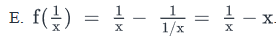Not equal to f(x).

QUESTION: 12

If f(x) = x + x2, is f(a+1) – f(a) divisible by 4, where a is an integer > 0

(1) f(a) is divisible by 4

(2)  (-1)a < (-1)a+1

Solution:

Steps 1 & 2: Understand Question and Draw Inferences

The question wants us to know whether f(a+1) – f(a) is divisible by 4. Let’s simplify the expression given to us.

f(a+1) – f(a) = (a+1) + (a+1)2 – (a + a2)

Simplifying we get f(a+1) – f(a) = (a + 1 –a) + ((a+1)2 -a2))

• 1 + (a + 1 –a)(a+1+a) = 2 + 2a = 2(1+a)           … (using a2 – b2 = (a-b)(a+b))
• From the statement above we can conclude that the given expression is always divisible by 2.

Hence, for f(a+1) – f(a) to be divisible by 4, (1+a) must be divisible by 2, which means that a must be odd.

Step 3: Analyze Statement 1

Statement 1 says that f(a) is divisible by 4.

f(a) = a(1+a)

a(1+a) is the product of two consecutive integers. Therefore, one term out of a and 1+a will be even and the other will be odd. The product of these two terms will be even and will always be divisible by 2.

But, we are given that a(1+a) is divisible by 4 also.

This can happen only if

a)      a is divisible by 4 or

b)      1+a is divisible by 4 or

c)       Both a and 1+a are divisible by 2

Case c) is ruled out since a and 1+a are consecutive terms. Therefore, they cannot be both even.

If a is divisible by 4, then a is even.

If 1+a is divisible by 4, then a is odd.

Thus, we cannot determine with confidence whether a is odd or not.

Since Statement 1 does not give us a unique answer, it is not sufficient.

Step 4: Analyze Statement 2

Statement 2 says that (-1)a < (-1)a+1

This is only possible if a is odd, implying that a+1 is even.

Thus, a is indeed odd.

Since statement 2 gives us a unique answer, it is sufficient to arrive at the conclusion.

Step 5: Analyze Both Statements Together (if needed)

Since statement 2 gave us a unique answer, this step is not needed.

QUESTION: 13

The function {x} is defined as the lowest odd integer greater than x. What is the value of {x}?

(1) -3.1 < x < -2.5

(2) x2 < 9

Solution:

Steps 1 & 2: Understand Question and Draw Inferences

Let’s understand the meaning of {x}. If x = 2, then the odd integers greater than 2 are 3, 5, etc. The lowest among them is 3. Therefore {2} = 3.

Step 3: Analyze Statement 1

If x is between -3.1 and -3, then {x} = -3. If x is between -3 and -2.5, then {x} = -1.

INSUFFICIENT.

Step 4: Analyze Statement 2

x2 < 9 only for values of x between -3 and 3.

If x = is between -3 and – 1, {x} = -1, if x is between -1 and 1, {x} = 1 and if x is between 1 and 3, {x} = 3.

Therefore, {x} can be -1, 1 or 3.

INSUFFICIENT.

Step 5: Analyze Both Statements Together (if needed)

When we combine statements (1) and (2),

We know that

-3.1 < x < -2.5

AND

-3 < x < 3

The common solution is: -3 < x < -2.5

For this set of values, {x} = -1.

SUFFICIENT.

QUESTION: 14

If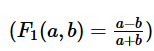and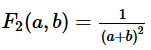then for positive integers (a, b) the maximum value of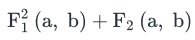is

Solution:

To find the maximum value, let’s first create a consolidated expression: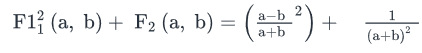Simplifying we get,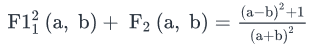The maximum value of the above expressions happens when a is much larger than b. Let’s say that a is 100 times larger than b, and b = 1 (the smallest allowed value for a positive integer);

hence a = 100.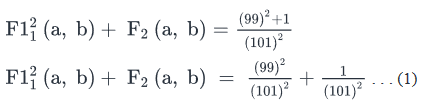The first term on right hand side is very close to 1, while the second time is very close to 0. Hence, we can say that the maximum value of the expression is close to but less than 1.

As the difference between a and b increases even further, the first term on the Right Hand Side of (1) will get still closer to 1, and the second term will approach zero, but the sum will still be lesser than 1.

Therefore, the correct answer choice is A

QUESTION: 15

The function SCI is defined as SCI(x, y) = z, where z is the sum of y consecutive positive integers starting from positive integer x. If a and n are positive integers, is SCI(a,n) divisible by n?

(1) 3n +2a  is not divisible by 2

(2) 3a +2n  is divisible by 2

Solution:

Steps 1 & 2: Understand Question and Draw Inferences

• SCI(x, y) = z, where z = x+ x+1 +x+2…….x+y-1 and x, y, z are integers > 0
• a, n are integers > 0

To Find: Is SCI(a, n) = nb, where quotient b is an integer?

• SCI(a, n) = a+ a+1….a+n -1
• The terms a, a + 1, a + 2…. a + n -1 are in arithmetic sequence with common difference  = 1
• SCI(a, n) = Sum of an arithmetic sequence =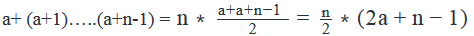• For SCI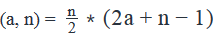to be divisible by n,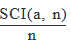should be an integer.
• That is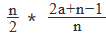should be an integer
• That is,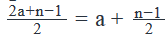should be an integer, i.e. n -1 should be divisible by 2.
• For n -1 to be divisible by 2, n-1 = even, i.e. n = odd.
• Thus, if n is odd SCI(a, n) is divisible by n, else it is not. Hence, we need to find the even-odd nature of n.

Step 3: Analyze Statement 1 independently

(1) 3n +2a  is not divisible by 2

• As 3n + 2a is not divisible by 2, 3n + 2a is odd
• 3n +2a = odd
• 3n + even = odd
• 3n = odd
• n = odd
• Since we know the even-odd nature of n, the statement is sufficient to answer.

Step 4: Analyze Statement 2 independently

(2) 3a +2n  is divisible by 2

• As 3a + 2n is divisible by 2, 3a + 2n is even
• 3a + 2n = even
• 3a + even = even
• 3a = even
• Does not tell us anything about the even-odd nature of n.